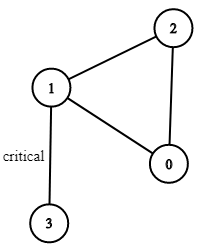##### Welcome to Subscribe On Youtube

Formatted question description: https://leetcode.ca/all/1192.html

# 1192. Critical Connections in a Network

Hard

## Description

There are n servers numbered from 0 to n-1 connected by undirected server-to-server connections forming a network where connections[i] = [a, b] represents a connection between servers a and b. Any server can reach any other server directly or indirectly through the network.

A critical connection is a connection that, if removed, will make some server unable to reach some other server.

Return all critical connections in the network in any order.

Example 1:Input: n = 4, connections = [[0,1],[1,2],[2,0],[1,3]]

Output: [[1,3]]

Explanation: [[3,1]] is also accepted.

Constraints:

• 1 <= n <= 10^5
• n-1 <= connections.length <= 10^5
• connections[i] != connections[i]
• There are no repeated connections.

## Solution

Use Tarjan algorithm. Maintain two arrays times and roots that stores the timesteps each node is met and the timesteps of the root of each connected component. Do depth first search starting from node 0.

Each time a new node is met, update the node’s times and roots value as the current timestep, and increase the timestep by 1. For each adjacent node of the current node, if it has not been visited, do depth first search from the adjacent node and update the current node’s roots value, and if the adjacent node’s roots value is greater than the current node’s times value, the connection of the current node and the adjacent node is a critical connection, so add it to the list of critical connections. Otherwise, update the current node’s roots value using the adjacent node’s times value (keeping the minimum).

Finally, return the critical connections.

• class Solution {
int[] times;
int[] roots;
int time;

public List<List<Integer>> criticalConnections(int n, List<List<Integer>> connections) {
times = new int[n];
roots = new int[n];
time = 0;
Arrays.fill(times, -1);
Arrays.fill(roots, -1);
Map<Integer, List<Integer>> edgesMap = new HashMap<Integer, List<Integer>>();
for (List<Integer> connection : connections) {
int node1 = connection.get(0), node2 = connection.get(1);
List<Integer> list1 = edgesMap.getOrDefault(node1, new ArrayList<Integer>());
List<Integer> list2 = edgesMap.getOrDefault(node2, new ArrayList<Integer>());
edgesMap.put(node1, list1);
edgesMap.put(node2, list2);
}
List<List<Integer>> criticalConnections = new ArrayList<List<Integer>>();
depthFirstSearch(edgesMap, 0, criticalConnections, -1);
return criticalConnections;
}

public void depthFirstSearch(Map<Integer, List<Integer>> edgesMap, int node, List<List<Integer>> criticalConnections, int parent) {
times[node] = time;
roots[node] = time;
time++;
List<Integer> adjacentList = edgesMap.getOrDefault(node, new ArrayList<Integer>());
continue;
} else
}
}
}

• class Solution {
public:
int count = 0;
vector<int> dfn, low;
vector<vector<int>> graph;
vector<vector<int>> res;
void tarjan(int u, int fa) {
dfn[u] = low[u] = ++count;
for (auto& v : graph[u]) {
if (v == fa)
continue;
if (!dfn[v]) {
tarjan(v, u);
low[u] = min(low[u], low[v]);
if (dfn[u] < low[v])
res.push_back({u, v});
} else {
low[u] = min(dfn[v], low[u]);
}
}
}

vector<vector<int>> criticalConnections(int n, vector<vector<int>>& connections) {
dfn.resize(n);
low.resize(n);
graph.resize(n);
for (auto& edge : connections) {
graph[edge].push_back(edge);
graph[edge].push_back(edge);
}
for (int i = 0; i < n; i++) {
if (!dfn[i])
tarjan(i, -1);
}
return res;
}
};


• class Solution:
def criticalConnections(
self, n: int, connections: List[List[int]]
) -> List[List[int]]:
def tarjan(a: int, fa: int):
nonlocal now
now += 1
dfn[a] = low[a] = now
for b in g[a]:
if b == fa:
continue
if not dfn[b]:
tarjan(b, a)
low[a] = min(low[a], low[b])
if low[b] > dfn[a]:
ans.append([a, b])
else:
low[a] = min(low[a], dfn[b])

g = [[] for _ in range(n)]
for a, b in connections:
g[a].append(b)
g[b].append(a)

dfn =  * n
low =  * n
now = 0
ans = []
tarjan(0, -1)
return ans


• func criticalConnections(n int, connections [][]int) (ans [][]int) {
now := 0
g := make([][]int, n)
dfn := make([]int, n)
low := make([]int, n)
for _, e := range connections {
a, b := e, e
g[a] = append(g[a], b)
g[b] = append(g[b], a)
}
var tarjan func(int, int)
tarjan = func(a, fa int) {
now++
dfn[a], low[a] = now, now
for _, b := range g[a] {
if b == fa {
continue
}
if dfn[b] == 0 {
tarjan(b, a)
low[a] = min(low[a], low[b])
if low[b] > dfn[a] {
ans = append(ans, []int{a, b})
}
} else {
low[a] = min(low[a], dfn[b])
}
}
}
tarjan(0, -1)
return
}

func min(a, b int) int {
if a < b {
return a
}
return b
}

• function criticalConnections(n: number, connections: number[][]): number[][] {
let now: number = 0;
const g: number[][] = Array(n)
.fill(0)
.map(() => []);
const dfn: number[] = Array(n).fill(0);
const low: number[] = Array(n).fill(0);
for (const [a, b] of connections) {
g[a].push(b);
g[b].push(a);
}
const ans: number[][] = [];
const tarjan = (a: number, fa: number) => {
dfn[a] = low[a] = ++now;
for (const b of g[a]) {
if (b === fa) {
continue;
}
if (!dfn[b]) {
tarjan(b, a);
low[a] = Math.min(low[a], low[b]);
if (low[b] > dfn[a]) {
ans.push([a, b]);
}
} else {
low[a] = Math.min(low[a], dfn[b]);
}
}
};
tarjan(0, -1);
return ans;
}